# Active Contours using Level Sets¶

Important: Please read the installation page for details about how to install the toolboxes. $\newcommand{\dotp}{\langle #1, #2 \rangle}$ $\newcommand{\enscond}{\lbrace #1, #2 \rbrace}$ $\newcommand{\pd}{ \frac{ \partial #1}{\partial #2} }$ $\newcommand{\umin}{\underset{#1}{\min}\;}$ $\newcommand{\umax}{\underset{#1}{\max}\;}$ $\newcommand{\umin}{\underset{#1}{\min}\;}$ $\newcommand{\uargmin}{\underset{#1}{argmin}\;}$ $\newcommand{\norm}{\|#1\|}$ $\newcommand{\abs}{\left|#1\right|}$ $\newcommand{\choice}{ \left\{ \begin{array}{l} #1 \end{array} \right. }$ $\newcommand{\pa}{\left(#1\right)}$ $\newcommand{\diag}{{diag}\left( #1 \right)}$ $\newcommand{\qandq}{\quad\text{and}\quad}$ $\newcommand{\qwhereq}{\quad\text{where}\quad}$ $\newcommand{\qifq}{ \quad \text{if} \quad }$ $\newcommand{\qarrq}{ \quad \Longrightarrow \quad }$ $\newcommand{\ZZ}{\mathbb{Z}}$ $\newcommand{\CC}{\mathbb{C}}$ $\newcommand{\RR}{\mathbb{R}}$ $\newcommand{\EE}{\mathbb{E}}$ $\newcommand{\Zz}{\mathcal{Z}}$ $\newcommand{\Ww}{\mathcal{W}}$ $\newcommand{\Vv}{\mathcal{V}}$ $\newcommand{\Nn}{\mathcal{N}}$ $\newcommand{\NN}{\mathcal{N}}$ $\newcommand{\Hh}{\mathcal{H}}$ $\newcommand{\Bb}{\mathcal{B}}$ $\newcommand{\Ee}{\mathcal{E}}$ $\newcommand{\Cc}{\mathcal{C}}$ $\newcommand{\Gg}{\mathcal{G}}$ $\newcommand{\Ss}{\mathcal{S}}$ $\newcommand{\Pp}{\mathcal{P}}$ $\newcommand{\Ff}{\mathcal{F}}$ $\newcommand{\Xx}{\mathcal{X}}$ $\newcommand{\Mm}{\mathcal{M}}$ $\newcommand{\Ii}{\mathcal{I}}$ $\newcommand{\Dd}{\mathcal{D}}$ $\newcommand{\Ll}{\mathcal{L}}$ $\newcommand{\Tt}{\mathcal{T}}$ $\newcommand{\si}{\sigma}$ $\newcommand{\al}{\alpha}$ $\newcommand{\la}{\lambda}$ $\newcommand{\ga}{\gamma}$ $\newcommand{\Ga}{\Gamma}$ $\newcommand{\La}{\Lambda}$ $\newcommand{\si}{\sigma}$ $\newcommand{\Si}{\Sigma}$ $\newcommand{\be}{\beta}$ $\newcommand{\de}{\delta}$ $\newcommand{\De}{\Delta}$ $\newcommand{\phi}{\varphi}$ $\newcommand{\th}{\theta}$ $\newcommand{\om}{\omega}$ $\newcommand{\Om}{\Omega}$

This tour explores image segementation using level set methods.

In :
options(warn=-1) # turns off warnings, to turn on: "options(warn=0)"

library(imager)
library(png)

for (f in list.files(path="nt_toolbox/toolbox_general/", pattern="*.R")) {
source(paste("nt_toolbox/toolbox_general/", f, sep=""))
}

for (f in list.files(path="nt_toolbox/toolbox_signal/", pattern="*.R")) {
source(paste("nt_toolbox/toolbox_signal/", f, sep=""))
}

source("nt_toolbox/toolbox_wavelet_meshes/meshgrid.R")
options(repr.plot.width=3.5, repr.plot.height=3.5)


## Managing level set functions¶

In the level set formalism, the evolution of some curve $(\ga(t))_{t=0}^1$ is computed by evolving the zero level of a function $\phi : \RR^2 \rightarrow \RR$ $$\enscond{\ga(s)}{ s \in [0,1] } = \enscond{x \in \RR^2}{\phi(x)=0}.$$ This corresponds to replacing the parameteric representation $\ga$ of the curve by an implicit representation. This requires an additional dimension (and hence more storage) but ease the handling of topological change of the curve during the evolution.

Discretazion size $n \times n$ of the domain $[0,1]^2$.

In :
n <- 200
grid <- meshgrid_2d(1:n, 1:n)
Y <- grid$X ; X <- grid$Y


One can create a circular shape by using the signed distance function to a circle $$\phi_1(x) = \sqrt{ (x_1-c_1)^2 + (x_2-c_2)^2 } - r$$ where $r>0$ is the radius and $c \in \RR^2$ the center.

Radius $r$.

In :
r <- n/3.


Center $c$.

In :
c <- c(r,r) + 10


Distance function $\phi_1$.

In :
phi1 <- sqrt((X-c)**2 + (Y-c)**2) - r


Exercise 1

Load a square shape $\phi_2$ at a different position for the center.

In :
source("nt_solutions/segmentation_3_snakes_levelset/exo1.R")

In :
## Insert your code here.


Display the curves associated to $\phi_1$ and $\phi_2$.

In :
options(repr.plot.width=7, repr.plot.height=3.5)
par(mfrow=c(1,2))

plot_levelset(phi1, lw=2)

plot_levelset(phi2, lw=2)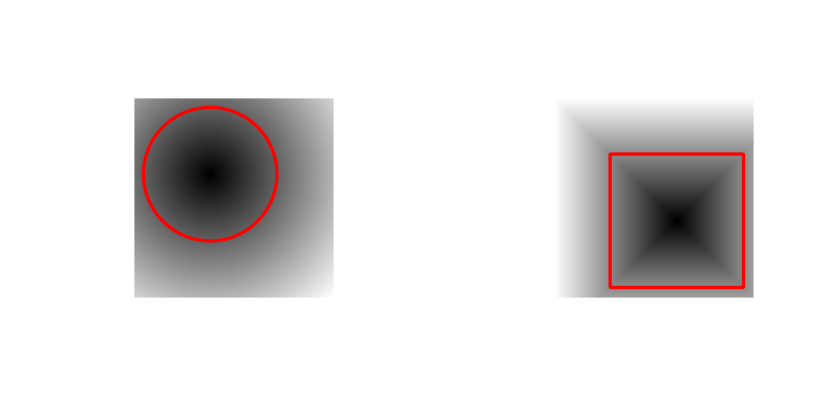Exercise 2

Compute the intersection and the union of the two shapes. Store the union in $\phi_0$ (phi0) that we will use in the remaining part of the tour.

In :
source("nt_solutions/segmentation_3_snakes_levelset/exo2.R")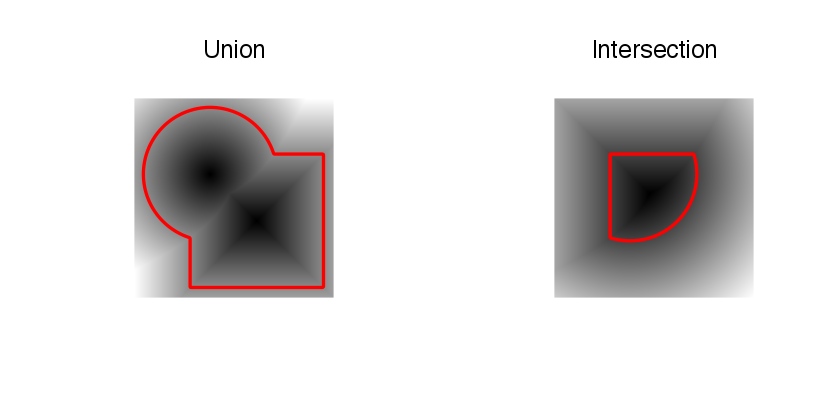In :
## Insert your code here.


## Mean Curvature Motion.¶

The mean curvature motion corresponds to the minimizing flow of the length of the curve $$\int_0^1 \norm{\ga'(s)} d s.$$

It is implemeted in a level set formalism by a familly $\phi_t$ of level set function parameterized by an artificial time $t \geq 0$, that satisfies the following PDE $$\pd{\phi_t}{t} = -G(\phi_t) \qwhereq G(\phi) = -\norm{\nabla \phi} \text{div} \pa{ \frac{\nabla \phi}{\norm{\nabla \phi}} }$$ and where $\nabla \phi_t(x) \in \RR^2$ is the spacial gradient.

This flow is computed using a gradient descent $\phi^{(0)} = \phi_0$ and $$\phi^{(\ell+1)} = \phi^{(\ell)} - \tau G(\phi^{(\ell)}),$$ where $\tau>0$ is small enough time step.

Maximum time of the evolution $0 \leq t \leq t_{\max}$.

In :
Tmax <- 200


Time step $\tau>0$ (should be small).

In :
tau <- .5


Number of iterations.

In :
niter <- as.integer(Tmax/tau)


Initial shape $\phi^{(0)}$ at $t=0$.

In :
phi <- phi0


We now compute the right hand side of the evolution equation.

Compute the gradient $\nabla \phi$. We use centered differences for the discretization of the gradient.

In :
g0 <- grad(phi, order=2)


Norm $\norm{\nabla \phi}$ of the gradient.

In :
eps <- .Machine$double.eps d <- pmax( eps*array(1,c(n,n)), sqrt(apply(g0**2, c(1,2), sum)) )  Normalized gradient. In : g <- g0/array(rep(d,2), c(dim(d),2))  The curvature term. In : K <- - d*div(g[,,1], g[,,2], order=2)  Perform one step of the gradient descent. In : phi <- phi - tau*K  Exercise 3 Implement the mean curvature motion. In : options(repr.plot.width=7, repr.plot.height=7) source("nt_solutions/segmentation_3_snakes_levelset/exo3.R")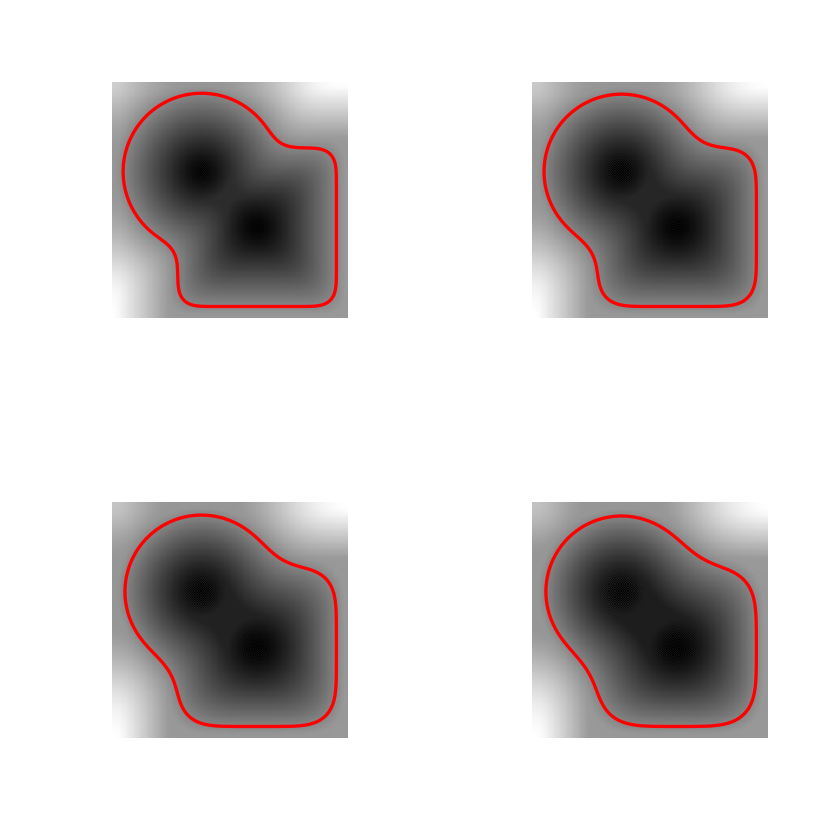In : ## Insert your code here.  ## Edge-based Segmentation with Geodesic Active Contour¶ Geodesic active contours compute loval minimum of a weighted geodesic distance that attract the curve toward the features of the background image. Note: these active contours should not be confounded with the geodesic shortest paths, that are globally minimizing geodesics between two points. Here the active contour is a close curve progressively decreasing a weighted geodesic length that is only a local minimum (the global minimum would be a single point). Size of the image. In : n <- 200  First we load an image$f_0 \in \RR^{n \times n}$to segment. In : f0 <- rescale(as.matrix(load_image("nt_toolbox/data/cortex.png", n)))  Given a background image$f_0$to segment, one needs to compute an edge-stopping function$W$. It should be small in area of high gradient, and high in area of large gradient. We use here $$W(x) = \al + \frac{\be}{\epsilon + d(x) } \qwhereq d = \norm{\nabla f_0} \star h_a,$$ and where$h_a$is a blurring kernel of size$a>0$. Compute the magnitude of the gradient$d_0(x) = \norm{\nabla f_0(x)}$. In : g <- grad(f0, order=2) d0 <- sqrt(apply(g**2, c(1,2), sum))  Blur size$a$. In : a <- 5  Compute the blurring$d = d_0 \star h_a$. In : d <- perform_blurring(d0, c(a), bound="per")   21 21  Parameter$\epsilon>0$. In : epsilon <- 1e-1  We set the$\al$and$\be$parameters to adjust the overall values of$W$(equivalently we use the function rescale). In : W <- 1./(epsilon + d) W <- rescale(-W, 0.1, 1)  Display it. In : options(repr.plot.width=7, repr.plot.height=3.5) imageplot(f0, "Image to segment", c(1,2,1)) imageplot(W, "Weight", c(1,2,2))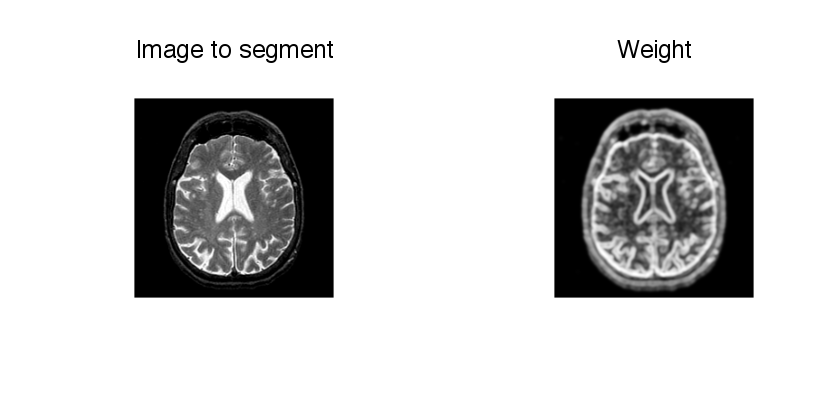Exercise 4 Compute an initial shape$\phi_0$at time$t=0$, for instance a centered square. In : source("nt_solutions/segmentation_3_snakes_levelset/exo4.R")  In : ## Insert your code here.  Display it. In : options(repr.plot.width=3.5, repr.plot.height=3.5) plot_levelset(phi0, f0, lw=2)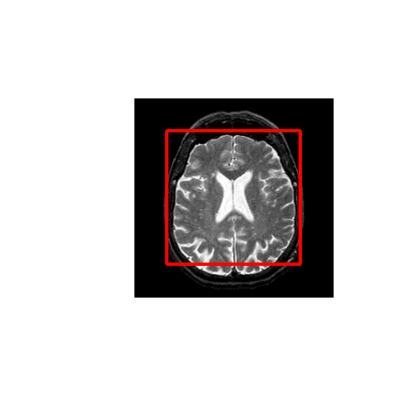The geodesic active contour minimizes a weighted length of curve $$\umin{\ga} \int_0^1 \norm{\ga'(s)} W(\ga(s)) d s$$ The level set implementation of the gradient descent of this energy reads $$\pd{\phi_t}{t} = G(\phi_t) \qwhereq G(\phi) = -\norm{\nabla \phi} \text{div}\pa{ W \frac{\nabla \phi}{\norm{\nabla \phi}} }$$ This is implemented using a gradient descent scheme. $$\phi^{(\ell+1)} = \phi^{(\ell)} - \tau G(\phi^{(\ell)}),$$ where$\tau>0$is small enough. Gradient step size$\tau>0$. In : tau <- .4  Final time and number of iteration of the algorithm. In : Tmax <- 1500 niter <- as.integer(Tmax/tau)  Initial distance function$\phi^{(0)}=\phi_0$. In : phi <- phi0  Note that we can re-write the gradient of the energy as $$G(\phi) = -W \norm{\nabla \phi} \text{div} \pa{ \frac{\nabla \phi}{\norm{\nabla \phi}} } - \dotp{\nabla W}{\nabla \phi}$$ Pre-compute once for all$\nabla W$. In : gW <- grad(W, order=2)  Exercise 5 Compute and store in G the gradient$G(\phi)$(right hand side of the PDE) using the current value of the distance function$\phi$. In : source("nt_solutions/segmentation_3_snakes_levelset/exo5.R")  In : ## Insert your code here.  Do the descent step. In : phi <- phi - tau*G  Exercise 6 Implement the geodesic active contours gradient descent. In : options(repr.plot.width=7, repr.plot.height=7) source("nt_solutions/segmentation_3_snakes_levelset/exo6.R")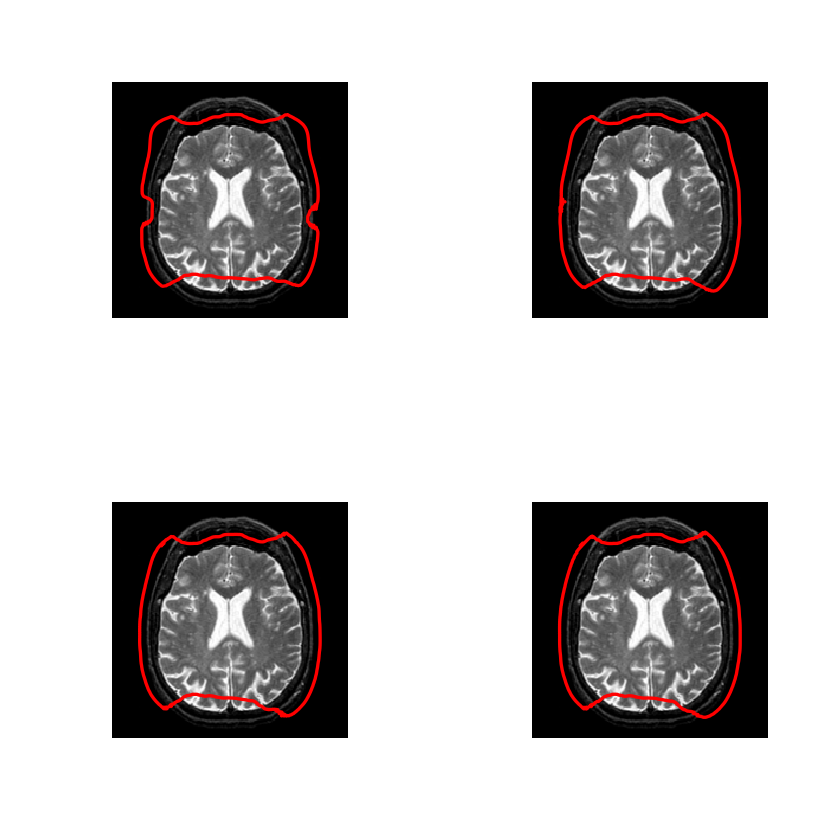In : ## Insert your code here.  ## Region-based Segmentation with Chan-Vese ¶ Chan-Vese active contours corresponds to a region-based energy that looks for a piecewise constant approximation of the image. The energy to be minimized is $$\umin{\phi} L(\phi) + \la \int_{\phi(x)>0} \abs{f_0(x)-c_1}^2 d x + \la \int_{\phi(x)<0} \abs{f_0(x)-c_2}^2 d x$$ where$L$is the length of the zero level set of$\phi$. Note that here$(c_1,c_2) \in \RR^2$are assumed to be known. Exercise 7 Compute an initial level set function$\phi_0$, stored in phi0, for instance many small circles. In : options(repr.plot.width=7, repr.plot.height=3.5) source("nt_solutions/segmentation_3_snakes_levelset/exo7.R")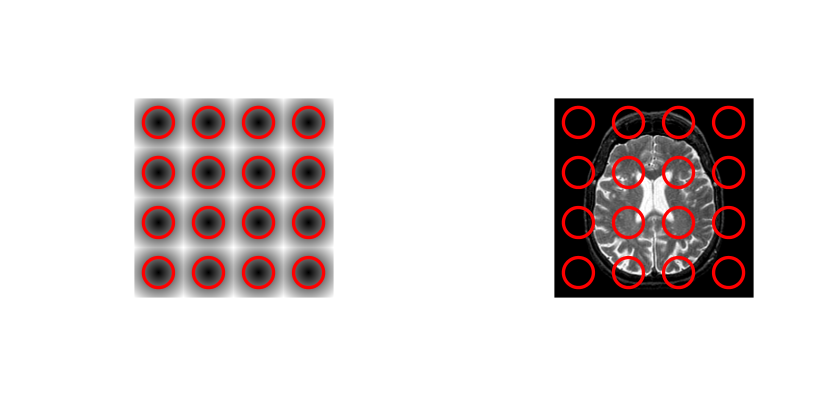In : ## Insert your code here.  Parameter$\la$In : lambd <- 2  Values for$c_1,c_2$In : c1 <- .7 c2 <- 0  Step size. In : tau <- .5  Number of iterations. In : Tmax <- 100 niter <- as.integer(Tmax/ tau)  Initial distance function$\phi_0$at time$t=0$. In : phi <- phi0  The minimizing flow for the CV energy reads $$\pd{\phi_t}{t} = - G(\phi_t)$$ where $$G(\phi) = • W \norm{\nabla \phi} \text{div}\pa{ \frac{\nabla \phi}{\norm{\nabla \phi}} } + \la (f_0-c_1)^2 - \la (f_0-c_2)^2.$$ Exercise 8 Compute this gradient$G(\phi)\$ using the current value of the distance function

In :
source("nt_solutions/segmentation_3_snakes_levelset/exo8.R")

In :
## Insert your code here.


Do a descent step.

In :
phi <- phi + tau*G


Exercise 9

options(repr.plot.width=7, repr.plot.height=7)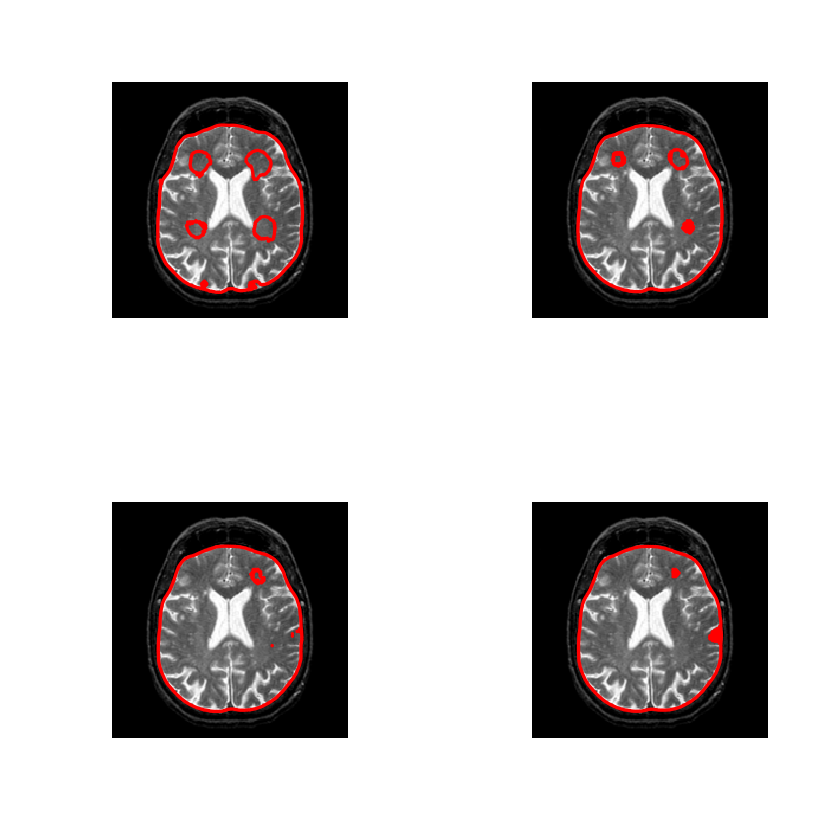## Insert your code here.